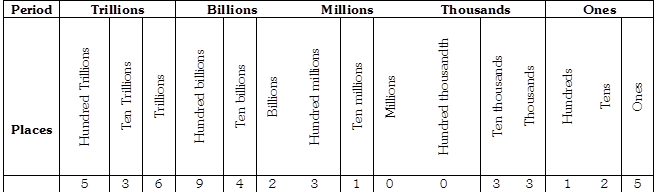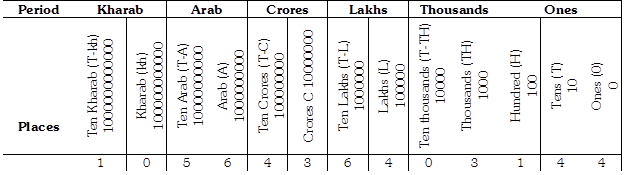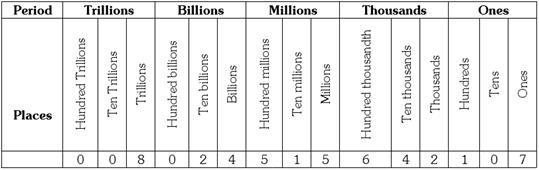Courses

# Test : Numbers - 1

## 10 Questions MCQ Test Mathematics for Class 5: NCERT | Test : Numbers - 1

Description
This mock test of Test : Numbers - 1 for Class 5 helps you for every Class 5 entrance exam. This contains 10 Multiple Choice Questions for Class 5 Test : Numbers - 1 (mcq) to study with solutions a complete question bank. The solved questions answers in this Test : Numbers - 1 quiz give you a good mix of easy questions and tough questions. Class 5 students definitely take this Test : Numbers - 1 exercise for a better result in the exam. You can find other Test : Numbers - 1 extra questions, long questions & short questions for Class 5 on EduRev as well by searching above.
QUESTION: 1

Solution:
QUESTION: 2

Solution:
QUESTION: 3

### Name the number indicated in the following place value chart.Solution:
QUESTION: 4

Name the number, indicated in the Indian place value chart, as per the international system of numeration.Solution:
QUESTION: 5

Name the number, indicated in the International place value chart, as per the Indian system of numeration.Solution:
QUESTION: 6

Find the place value of 6 in the numeral which is 5453445565 more than 64445563230.

Solution:
QUESTION: 7

Find the successor of the number which is equal to the product of 4354565 and 5798.

Solution:
QUESTION: 8

Steve has 3 million and 4 hundred thousand rupees. What is the worth of his money as per Indian system of numeration?

Solution:
QUESTION: 9

In the number 2, 75, 465, what is the difference between the place value of 5?

Solution:
QUESTION: 10

Find the predecessor of the number which is 454412 less than X. X is the seven digit smallest number.

Solution: